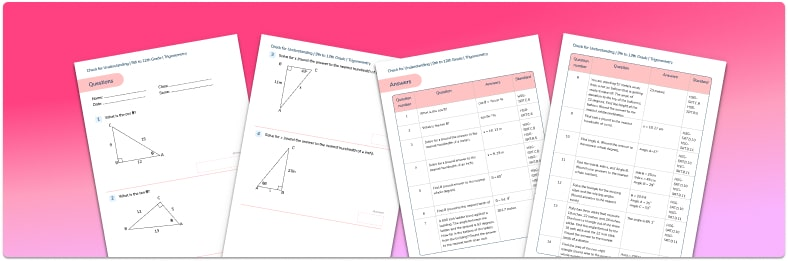Math teaching support you can trustone-on-one tutoring sessionsschools supported

[FREE] Fun Math Games & Activities Packs

Always on the lookout for fun math games and activities in the classroom? Try our ready-to-go printable packs for students to complete independently or with a partner!

# 15 Trigonometry Questions And Practice Problems To Do With High Schoolers

Trigonometry questions address the relationship between the angles of a triangle and the lengths of the sides. By using our knowledge of the rules of trigonometry and trigonometric functions, we can calculate missing angles or sides when we have been given some of the information.

Here we’ve provided 15 trigonometry questions to provide students with practice at the various sorts of trigonometry problems exam style questions you can expect them to encounter during high school.Trigonometry Check for Understanding Quiz

Use this quiz to check your 9-12th grade students’ understanding of Trigonometry.Download Free Now!

### Trigonometry in the real world

Trigonometry is used by architects, engineers, astronomers, crime scene investigators, flight engineers and many others.

### Trigonometry in high school

In trigonometry we learn about the sine function, tangent function and cosine function. These trig functions are abbreviated as sin, cos and tan. We can use these to calculate sides and angles in right triangles. Later, students will be applying this to a variety of situations as well as learning the exact values of sin, cos and tan for certain angles.

Students learn about trigonometric ratios: the law of sines, law of cosines, a new formula for the area of a triangle and applying trigonometric theorems to 3D shapes.

Trigonometry for more senior high school students will introduce the reciprocal trig functions, cotangent, secant and cosecant, but you don’t have to worry about these right now!

### How to answer trigonometry questions

The way to answer trigonometry questions depends on whether it is a right triangle or not.

#### How to answer trigonometry questions: right triangles

If your question involves a right triangle, you can apply the trigonometric ratios of:

sin θ = opposite/hypotenuse

The acronym SOH CAH TOA is used so that you can remember which ratio to use.

1. Establish that it is a right triangle.

2. Label the opposite side (opposite the given angle), the adjacent side (next to the angle), and the hypotenuse (longest side opposite the right angle).

3. Use the following triangles to help us decide which calculation to do:

#### How to answer trigonometry questions – non-right triangles

If the triangle is not a right triangle then we need to use the sine rule or the cosine rule.

There is also a formula we can use for the area of a triangle, which does not require us to know the base and height of the triangle.

1. Establish that it is not a right triangle.
2. Label the sides of the triangle using lowercase a, b, c.
3. Label the angles of the triangle using upper case A, B and C.
4. Opposite sides and angles should use the same letter, for example, angle A is opposite to side a.

### Trigonometry questions

Trigonometry questions focus on understanding trigonometric identities,  sin, cos and tan ratios (SOHCAHTOA) to calculate missing sides and angles in right triangles. Questions may also require learners to think back to their knowledge on pythagorean theorem.

#### Trigonometry questions – missing side

1. A zip wire runs between two posts, 25m apart. The zip wire is at an angle of 10∘ to the horizontal. Calculate the length of the zip wire.

[image]

25.4m

144.0m

141.8m

24.6m

2. A surveyor wants to know the height of a skyscraper. He places his inclinometer on a tripod 1m from the ground. At a distance of 50m from the skyscraper, he records an angle of elevation of 82∘.

What is the height of the skyscraper? Give your answer to one decimal place.

[image]

355.8m

7.0m

356.8m

49.5m

3. Triangle ABC is isosceles. Work out the height of triangle ABC.

[image]

6cm

17.4cm

34.9cm

2.1cm

#### Trigonometry questions – missing angles

4. A builder is constructing a roof. The wood he is using for the sloped section of the roof is 4m long and the peak of the roof needs to be 2m high. What angle should the piece of wood make with the base of the roof?

[image]

26.6∘

60∘

0.008∘

30∘

5. A ladder is leaning against a wall. The ladder is 1.8m long and the bottom of the ladder is 0.5m from the base of the wall. To be considered safe, a ladder must form an angle of between 70∘ and 80∘ with the floor. Is this ladder safe?

[images]

Yes

No

Not enough information

6. A helicopter flies 40km east followed by 105km south. On what bearing must the helicopter fly to return home directly?

21∘

201∘

159∘

339∘

Looking for more high school math questions and word problems?

Try these:

Do you have students who need extra support in math?
Give your students more opportunities to consolidate learning and practice skills through personalized math tutoring with their own dedicated online math tutor.

Each student receives differentiated instruction designed to close their individual learning gaps, and scaffolded learning ensures every student learns at the right pace. Lessons are aligned with your state’s standards and assessments, plus you’ll receive regular reports every step of the way.

Personalized one-on-one math tutoring programs are available for:

The content in this article was originally written by secondary school maths teacher Beki Christian and has since been revised and adapted for US schools by elementary math teacher Christi Kulesza.##### Beki Christian
Maths Teacher and Author
Beki has 11 years experience teaching secondary Maths. She is currently working as a Maths tutor as well as writing resources and blog posts for Third Space Learning.
x#### Trigonometry Check for Understanding Quiz

Use this quiz to check your 9-12th grade students’ understanding of Trigonometry.

#### Trigonometry Check for Understanding QuizUse this quiz to check your 9-12th grade students’ understanding of Trigonometry.